## Wednesday, 14 December 2011

### Mathematical induction and its consequences Part 1

Here we would concentrate in illustrating the case n = k + 1 assuming n = k is correct with n = 1 is correct.

1. Polynomials

Example. The most stupid M.I. in the world, showing that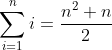.
In polynomial form M.I. is always easy: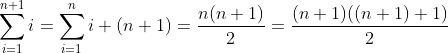so that the proof is complete.

Example 2.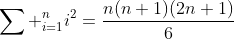Proof.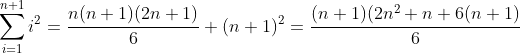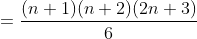which finishes the proof.

Exercise 1. Show that:
i)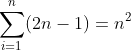, e.g.,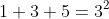ii)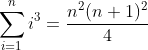In fact, there's no such a general form for sum of the n-th power of natrual number.

2. Divisibility and integral solution

Example 3.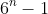is divisible by 5.
Let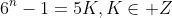, then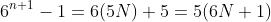.

In more complicated case, some of the grouping method may not work.

Example 4.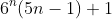is divisible by 25.
Let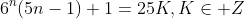, then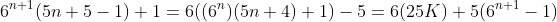where the last term is divisible by 25 by ex.3.

Example 5. (CSMO)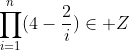for all positive integer n.
It seems to be very fresh to most M2 or even Pure student as you don't know the proposition to be set in order to prove the statement.Think inversely, the next product must be integer if the last one is a multiple, (and of course, an integer) of the next i, then the next term must be integer too.
Therefore the proposition is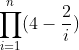is a multiple of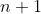.

Exercise 2. Show that
1)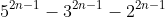is divisible by 15 by induction on n.
2) Finish the proof for ex. 5.

Challenge 1. It's given that the first 4 terms are 2,6,20,70,...
a) Give a general formula for the series with proof.
b) The series satisfies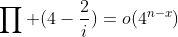, describe x.

The last example is from HKALE.

Example 6. (HKALE Pure 1998, I5) For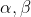as roots of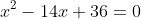, show that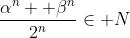for all natrual number n.

This is a 5-mark question and shouldn't be hard, or even fit perfectly for M2/Pure level, however we need another method of Mathematical Induction:

Theorem. Second principle of M.I. The proposition is true for all natrual n if:
1) n = 1,2,...i is true.
2) If n = j, (j+1), ... i+j is true, then n = i+j+1 is true.

in this case i = 2.
Assume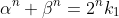and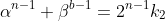.
By relationship between roots and equations, we have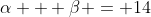,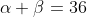.

Then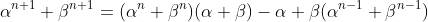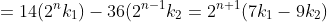The last term is trivially a positive integer.

Challenge 2. The recurrence relationship of the sequenceis shown. Find the general term of the sequence.

Exercise 3. Is it true that for x,y as roots of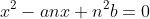satisfies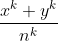is an integer? Prove your assertion. (a,b,n are natrual numbers.)

Read the rest of this passage:

Part II
Part III
Part IV